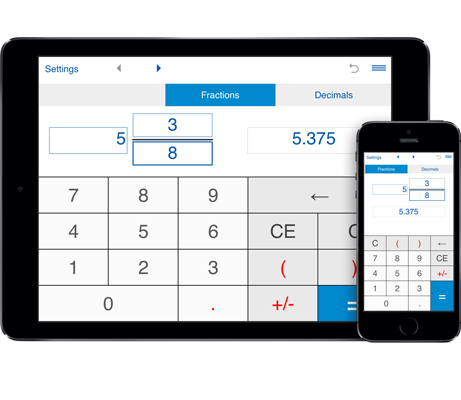# Fraction to decimal converter

This calculator will easily convert fractions to decimals and vice versa.
To convert proper and improper fractions and mixed numbers to decimals, select "fraction to decimal".

Fraction to Decimal Converter Settings

Number of decimal places

Round fraction to the nearest

## Convert Fractions to Decimals

1/2 = 0.5
1/3 = 0.33333333
2/3 = 0.66666667
1/4 = 0.25
2/4 = 0.5
3/4 = 0.75
1/5 = 0.2
2/5 = 0.4
3/5 = 0.6
4/5 = 0.8
1/6 = 0.16666667
2/6 = 0.33333333
3/6 = 0.5
4/6 = 0.66666667
5/6 = 0.83333333
1/7 = 0.14285714
2/7 = 0.28571429
3/7 = 0.42857143
4/7 = 0.57142857
5/7 = 0.71428571
6/7 = 0.85714286
1/8 = 0.125
2/8 = 0.25
3/8 = 0.375
4/8 = 0.5
5/8 = 0.625
6/8 = 0.75
7/8 = 0.875
1/9 = 0.11111111
2/9 = 0.22222222
3/9 = 0.33333333
4/9 = 0.44444444
5/9 = 0.55555556
6/9 = 0.66666667
7/9 = 0.77777778
8/9 = 0.88888889
1/10 = 0.1
2/10 = 0.2
3/10 = 0.3
4/10 = 0.4
5/10 = 0.5
6/10 = 0.6
7/10 = 0.7
8/10 = 0.8
9/10 = 0.9
1/11 = 0.09090909
1/12 = 0.08333333
2/12 = 0.16666667
3/12 = 0.25
4/12 = 0.33333333
5/12 = 0.41666667
6/12 = 0.5
7/12 = 0.58333333
8/12 = 0.66666667
9/12 = 0.75
10/12 = 0.83333333
11/12 = 0.91666667
1/13 = 0.07692308
1/14 = 0.07142857
1/15 = 0.06666667
1/16 = 0.0625
3/16 = 0.1875
5/16 = 0.3125
7/16 = 0.4375
9/16 = 0.5625
11/16 = 0.6875
13/16 = 0.8125
15/16 = 0.9375
1/17 = 0.05882353
1/18 = 0.05555556
1/19 = 0.05263158
1/20 = 0.05
1/25 = 0.04
1/32 = 0.03125
1/50 = 0.02
1/64 = 0.015625
1/100 = 0.01
1/125 = 0.008
1/128 = 0.0078125
1/250 = 0.004
1/256 = 0.00390625
1/500 = 0.002
1/1000 = 0.001
-
-
-
-
-
-
-
-
-
-
-
-
-

0.001 = 1/1000
0.002 = 1/500
0.003 = 3/1000
0.004 = 1/250
0.005 = 1/200
0.006 = 3/500
0.007 = 7/1000
0.008 = 1/125
0.009 = 9/1000
0.01 = 1/100
0.02 = 1/50
0.03 = 3/100
0.04 = 1/25
0.05 = 1/20
0.06 = 3/50
0.07 = 7/100
0.08 = 2/25
0.09 = 9/100
0.1 = 1/10
0.11 = 11/100
0.12 = 3/25
0.13 = 13/100
0.14 = 7/50
0.15 = 3/20
0.16 = 4/25
0.17 = 17/100
0.18 = 9/50
0.19 = 19/100
0.2 = 1/5
0.21 = 21/100
0.22 = 11/50
0.23 = 23/100
0.24 = 6/25
0.25 = 1/4
0.26 = 13/50
0.27 = 27/100
0.28 = 7/25
0.29 = 29/100
0.3 = 3/10
0.31 = 31/100
0.32 = 8/25
0.33 = 33/100
0.34 = 17/50
0.35 = 7/20
0.36 = 9/25
0.37 = 37/100
0.38 = 19/50
0.39 = 39/100
0.4 = 2/5
0.41 = 41/100
0.42 = 21/50
0.43 = 43/100
0.44 = 11/25
0.45 = 9/20
0.46 = 23/50
0.47 = 47/100
0.48 = 12/25
0.49 = 49/100
0.5 = 1/2
0.51 = 51/100
0.52 = 13/25
0.53 = 53/100
0.54 = 27/50
0.55 = 11/20
0.56 = 14/25
0.57 = 57/100
0.58 = 29/50
0.59 = 59/100
0.6 = 3/5
0.61 = 61/100
0.62 = 31/50
0.63 = 63/100
0.64 = 16/25
0.65 = 13/20
0.66 = 33/50
0.67 = 67/100
0.68 = 17/25
0.69 = 69/100
0.7 = 7/10
0.71 = 71/100
0.72 = 18/25
0.73 = 73/100
0.74 = 37/50
0.75 = 3/4
0.76 = 19/25
0.77 = 77/100
0.78 = 39/50
0.79 = 79/100
0.8 = 4/5
0.81 = 81/100
0.82 = 41/50
0.83 = 83/100
0.84 = 21/25
0.85 = 17/20
0.86 = 43/50
0.87 = 87/100
0.88 = 22/25
0.89 = 89/100
0.9 = 9/10
0.91 = 91/100
0.92 = 23/25
0.93 = 93/100
0.94 = 47/50
0.95 = 19/20
0.96 = 24/25
0.97 = 97/100
0.98 = 49/50
0.99 = 99/100
-
-
-
-
-
-
-
-
-
-
-
-

## Fraction Converter Calculator

This fraction to decimal calculator converts fractions (proper and improper fractions) and mixed numbers to decimals.

Input a fraction or a mixed number, click "=" and the fraction to decimal converter will easily convert a fraction into its decimal form.

## Converting fractions to decimals

### Fraction to Decimal Conversion

How to use the fraction to decimal converter:

#### Convert fractions to decimals:

To convert a fraction to a decimal using the fraction to decimal conversion calculator:

1. Select the "fraction to decimal" option;

2. Type the whole number (for mixed fractions), numerator and denominator of the fraction you want to convert;

3. Press "=".

The fraction you entered will be converted to a decimal.

Converting fractions to decimals:

The fraction converter will reduce a fraction and then convert it to a decimal number by dividing the numerator by the denominator.

Converting mixed fractions to decimals:

This fraction converter will convert a mixed number to an improper fraction, reduce that fraction if it can be reduced, then perform the division of the numerator by the denominator to find the decimal equivalent of the mixed number.

Fraction input:
You may enter positive and negative fractions.
The input fraction can be a proper or improper fraction, or a mixed number (mixed fraction).